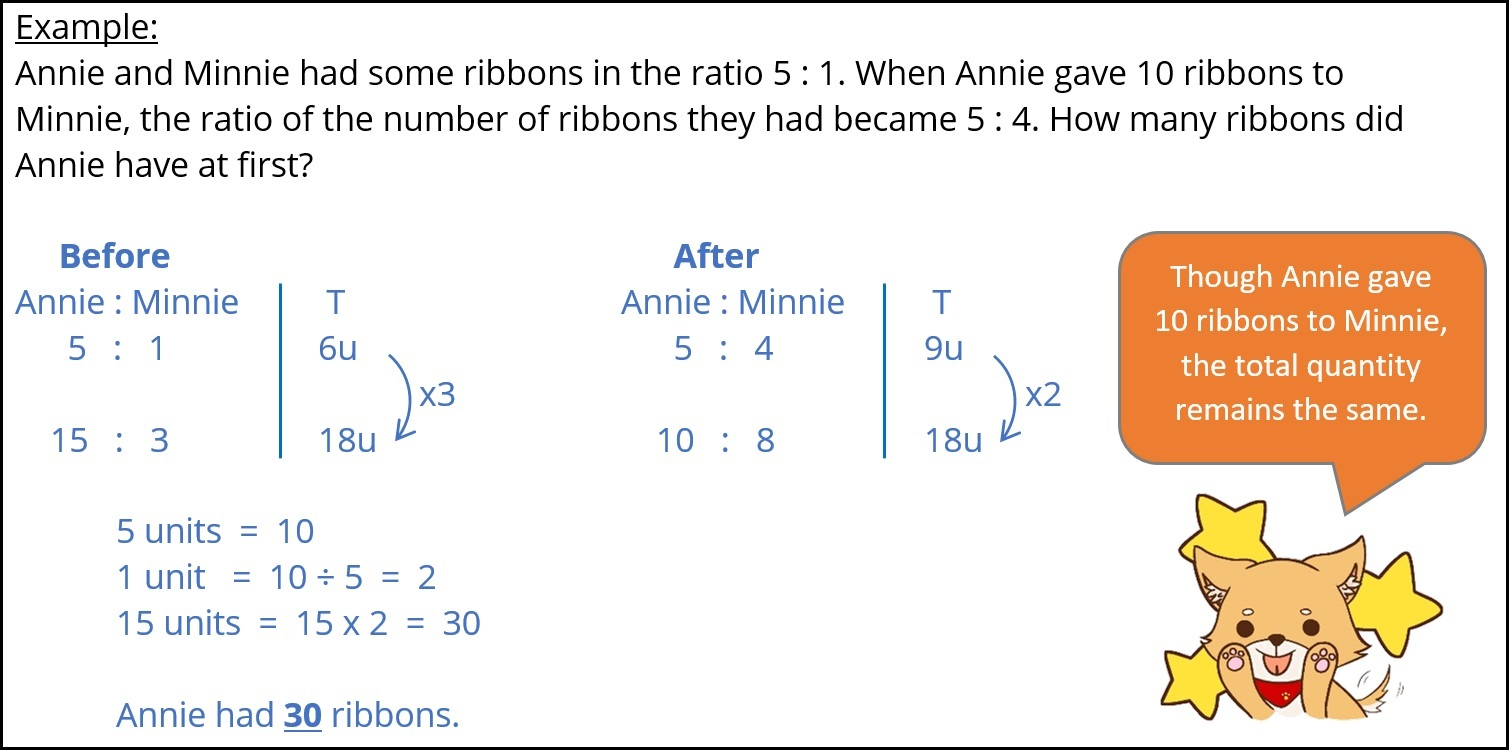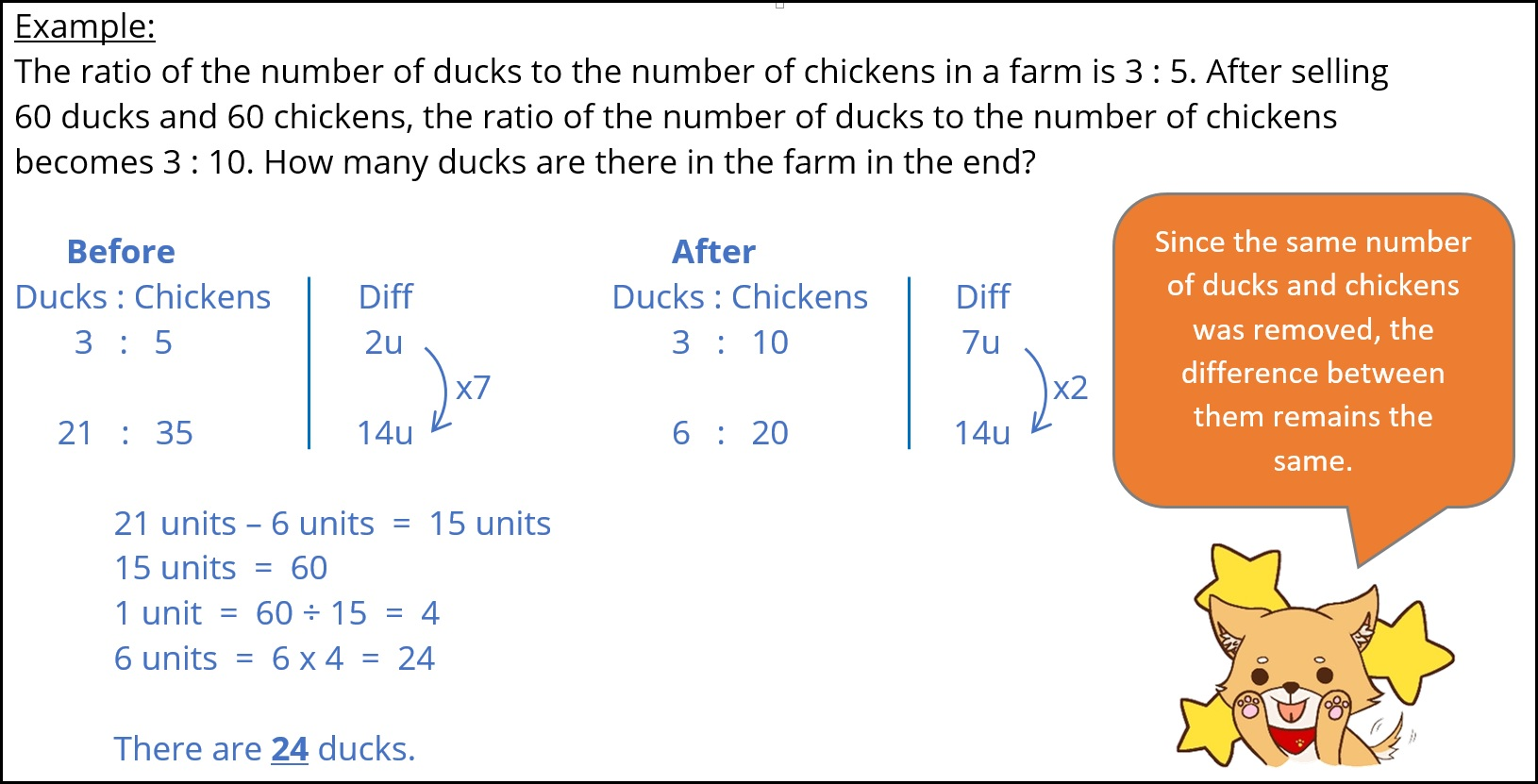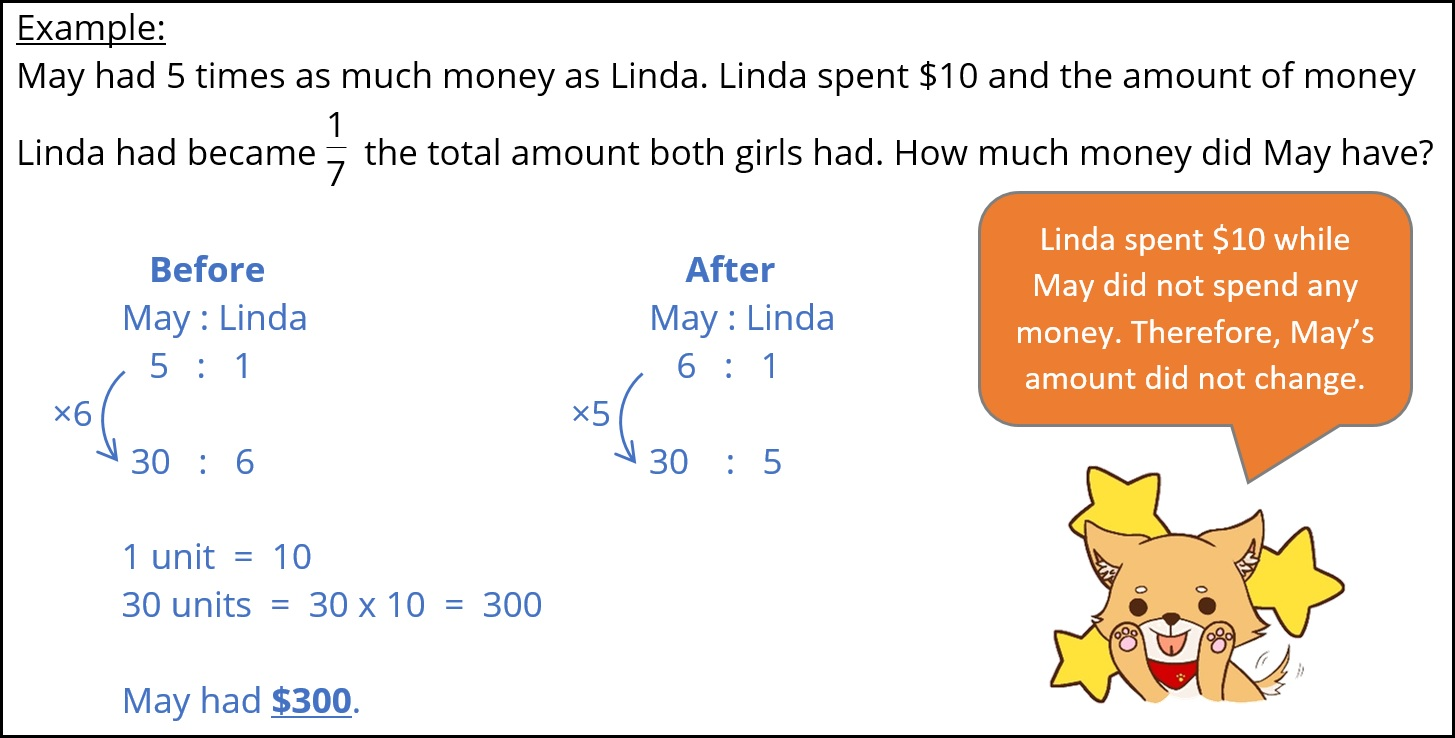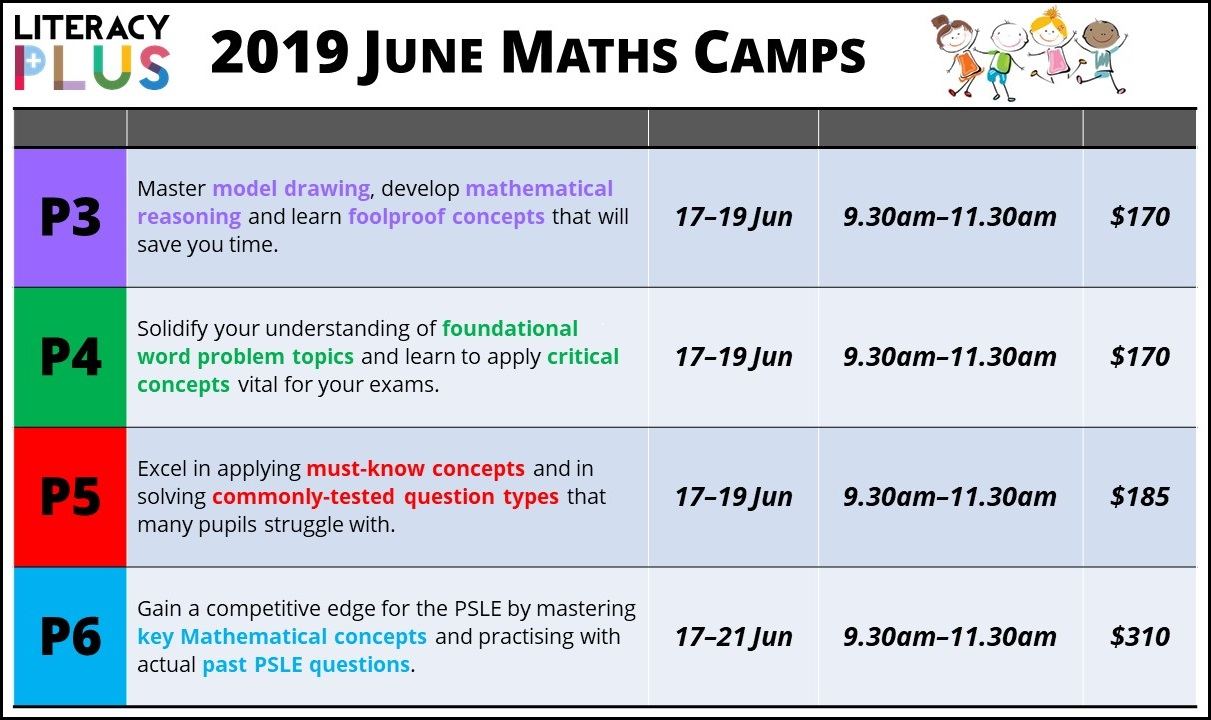# 3 Important Maths Concepts Your Child Must Know

Word problems get harder as pupils move up to Primary 5 and 6. Proper application of Maths concepts becomes even more critical to solving word problems. Pupils need to be able to recognise the underlying concepts that a word problem is testing and correctly pick out the essential information within the question. As a baseline, every pupil must know the following 3 concepts: Constant Total, Constant Difference and Constant Identity.

#### Concept #1: Constant Total

The principle of this concept is that the total quantity in the question needs to remain unchanged. For example, Person A can transfer a quantity to Person B or vice versa, but neither of these actions will change the total quantity in the question.For a video explanation of a P6 question on Constant Total, click here.

#### Concept #2: Constant Difference

For this concept to be applicable, an equal quantity needs to be added or taken away from the variables in the question. This ensures that the difference between the 2 variables remains unchanged. Hence the name Constant Difference.If your child is in Primary 4, models can be a useful visual tool to help illustrate this concept. For a video explanation on Constant Difference using models, click here.

#### Concept #3: Constant Identity

What characterises this concept is that the quantity of one variable must remain the same. In other words, there must neither be an increase nor a decrease in the quantity of that one variable.#### 2019 June Maths Camps